Power Functions

A power function is a function where the variable has a power greater than one. Graphs of power functions will vary depending upon the value of the exponent.

Power functions have equations in the form: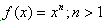. There are patterns in the shapes of the graphs of power functions. Power functions take two forms: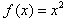and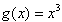. All power functions with even powers will graph similar toand power functions with odd powers will graph similar to.

See the table and graph of two basic power functions below.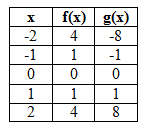f(x) = x2 g(x) = x3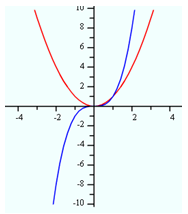Here are some situations that can be modeled by power functions:

• Area (area vs. length).
• Volume (volume vs. length).
• A falling object (height vs. time).